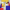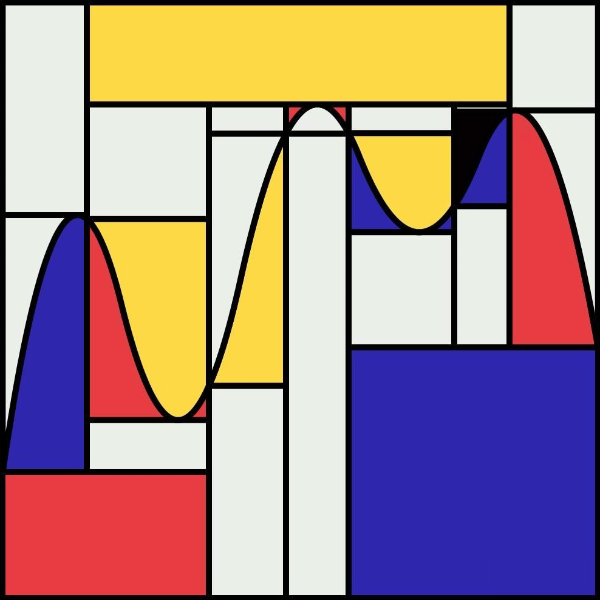# Replica analysis of overfitting in generalized linear models

Statistical methods that normally fail for very high-dimensional data can be rescued via mathematical tools from statistical physics.

Submitted to Journal of Physics A (2020)

T. Coolen, M. Sheikh, A. Mozeika, F. Aguirre-Lopez, F. AntenucciNearly all statistical inference methods were developed for the regime where the number N of data samples is much larger than the data dimension p. Inference protocols such as maximum likelihood (ML) or maximum a posteriori probability (MAP) are unreliable if p=O(N), due to overfitting. This limitation has for many disciplines with increasingly high-dimensional data become a serious bottleneck. We recently showed that in Cox regression for time-to-event data the overfitting errors are not just noise but take mostly the form of a bias, and how with the replica method from statistical physics once can model and predict this bias and the noise statistics. Here we extend our approach to arbitrary generalized linear regression models (GLM), with possibly correlated covariates. We analyse overfitting in ML/MAP inference without having to specify data types or regression models, relying only on the GLM form, and derive generic order parameter equations for the case of L2 priors. Second, we derive the probabilistic relationship between true and inferred regression coefficients in GLMs, and show that, for the relevant hyperparameter scaling and correlated covariates, the L2 regularization causes a predictable direction change of the coefficient vector. Our results, illustrated by application to linear, logistic, and Cox regression, enable one to correct ML and MAP inferences in GLMs systematically for overfitting bias, and thus extend their applicability into the hitherto forbidden regime p=O(N).#### Replica analysis of overfitting in generalized linear models

T. Coolen, M. Sheikh, A. Mozeika, F. Aguirre-Lopez, F. Antenucci

Sub. to Journal of Physics A

1 / 123 papers